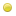Verify that the field "answer" is correctly completed.Snake_Posts: 188 Threads: 19 Joined: Dec 2013 Reputation: 11 2015-03-24, 02:31 PM (This post was last modified: 2015-03-24, 02:32 PM by Snake_. Edit Reason: small info. ) Hello.  If my thread is in bad forum, please move. My problem is in form. I downloaded from database questions from "Security Question [ACP]". This to the register page. I need use that my new page.  Ok, I have these questions, but how verify, that in field (input) "answer" entered the correct answer? This is code in my script (from member.php): ``````// Security Question \$questionbox = ''; if(\$mybb->settings['securityquestion']) { \$sid = generate_question(); \$query = \$db->query(" SELECT q.*, s.sid FROM ".TABLE_PREFIX."questionsessions s LEFT JOIN ".TABLE_PREFIX."questions q ON (q.qid=s.qid) WHERE q.active='1' AND s.sid='{\$sid}' "); if(\$db->num_rows(\$query) > 0) { \$question = \$db->fetch_array(\$query); \$refresh = ''; // Total questions \$q = \$db->simple_select('questions', 'COUNT(qid) as num', 'active=1'); \$num = \$db->fetch_field(\$q, 'num'); if(\$num > 1) { eval("\\$refresh = \"".\$templates->get("member_register_question_refresh")."\";"); } eval("\\$questionbox = \"".\$templates->get("member_register_question")."\";"); \$validator_extra = " \$('#answer').rules('add', { required: true, remote:{ url: 'xmlhttp.php?action=validate_question', type: 'post', dataType: 'json', data: { question: function () { return \$('#question_id').val(); }, my_post_key: my_post_key }, }, messages: { remote: '{\$lang->js_validator_no_security_question}' } });\n"; } } eval("\\$formularz = \"".\$templates->get("formularz")."\";");`````` Maybe you have other suggestions? Use: My script send on email information from the form. I use "security question" in order to security (not SPAM). Next I need verify correct answer. Regards. Sorry if sth not understand, but i wrote in translator..Destroy666Former Staff Posts: 5,525 Threads: 73 Joined: Feb 2012 Reputation: 582 2015-03-24, 02:45 PM (This post was last modified: 2015-03-24, 02:45 PM by Destroy666.) On PHP side: https://github.com/mybb/mybb/blob/featur...p#L249-294 For JS you need to load the Validate code just like the member_register template + include jscripts/question.js.My plugins / servicesSnake_Posts: 188 Threads: 19 Joined: Dec 2013 Reputation: 11 2015-03-24, 03:05 PM (This post was last modified: 2015-03-24, 03:05 PM by Snake_. Edit Reason: s ) Ok, I added this PHP code to file and to template "formularz": ````````````How now... Destroy666 Wrote:include jscripts/question.js....?Destroy666Former Staff Posts: 5,525 Threads: 73 Joined: Feb 2012 Reputation: 582 2015-03-24, 03:29 PM (This post was last modified: 2015-03-24, 03:29 PM by Destroy666.) Just load that script on the page in a firstMy plugins / servicesSnake_Posts: 188 Threads: 19 Joined: Dec 2013 Reputation: 11 2015-03-24, 05:01 PM Hmm... doesn't works... I loaded this file, but not results. Maybe I give me file and template. Template:  ``````

{\$questionbox} ``````And file (not all, because next is code form): ``````if(empty(\$_POST['submit'])) { // Security Question \$questionbox = ''; if(\$mybb->settings['securityquestion']) { \$question_id = \$db->escape_string(\$mybb->get_input('question_id')); \$answer = \$db->escape_string(\$mybb->get_input('answer')); \$query = \$db->query(" SELECT q.*, s.sid FROM ".TABLE_PREFIX."questionsessions s LEFT JOIN ".TABLE_PREFIX."questions q ON (q.qid=s.qid) WHERE q.active='1' AND s.sid='{\$question_id}' "); if(\$db->num_rows(\$query) > 0) { \$question = \$db->fetch_array(\$query); \$valid_answers = explode("\n", \$question['answer']); \$validated = 0; foreach(\$valid_answers as \$answers) { if(my_strtolower(\$answers) == my_strtolower(\$answer)) { \$validated = 1; } } if(\$validated != 1) { \$update_question = array( "incorrect" => \$question['incorrect'] + 1 ); \$db->update_query("questions", \$update_question, "qid='{\$question['qid']}'"); \$errors[] = "błędna odpowiedź"; } else { \$update_question = array( "correct" => \$question['correct'] + 1 ); \$db->update_query("questions", \$update_question, "qid='{\$question['qid']}'"); } \$db->delete_query("questionsessions", "sid='{\$sid}'"); } \$sid = generate_question(); \$query = \$db->query(" SELECT q.*, s.sid FROM ".TABLE_PREFIX."questionsessions s LEFT JOIN ".TABLE_PREFIX."questions q ON (q.qid=s.qid) WHERE q.active='1' AND s.sid='{\$sid}' "); if(\$db->num_rows(\$query) > 0) { \$question = \$db->fetch_array(\$query); \$answer = \$question['answer']; \$zla = "bledna  opdsadasd "; if(\$answer == FALSE) { \$answer = \$zla; } \$refresh = ''; // Total questions \$q = \$db->simple_select('questions', 'COUNT(qid) as num', 'active=1'); \$num = \$db->fetch_field(\$q, 'num'); if(\$num > 1) { eval("\\$refresh = \"".\$templates->get("member_register_question_refresh")."\";"); } eval("\\$questionbox = \"".\$templates->get("member_register_question")."\";"); \$validator_extra = " \$('#answer').rules('add', { required: true, remote:{ url: 'xmlhttp.php?action=validate_question', type: 'post', dataType: 'json', data: { question: function () { return \$('#question_id').val(); }, my_post_key: my_post_key }, }, messages: { remote: '{\$lang->js_validator_no_security_question}' } });\n"; } } eval("\\$formularz = \"".\$templates->get("formularz")."\";"); }``````I copied from member.php.Snake_Posts: 188 Threads: 19 Joined: Dec 2013 Reputation: 11 2015-03-25, 09:01 PM I updated code. This script is displayed on each page, but doesn't works. In browser console: ``Uncaught TypeError: Cannot read property 'nodeName' of null jquery:2`` Is ignored "member.php" - is not there currently. Any suggestions?Destroy666Former Staff Posts: 5,525 Threads: 73 Joined: Feb 2012 Reputation: 582 2015-03-25, 09:21 PM (This post was last modified: 2015-03-25, 09:26 PM by Destroy666.) 1. Why is the PHP validation executed when empty(\$_POST['submit'])? 2. Why is there no value for action attribute in form? 3. You also need to initiate validation for the whole
: \$("#formularz").validate(); before adding any rules.My plugins / services « Next Oldest | Next Newest »

Forum Jump:

Users browsing this thread: 1 Guest(s)